0%

# 离散对数问题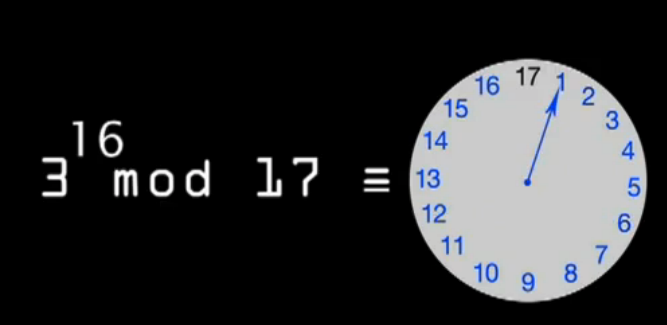$$3^{\color{red}{29}} \mathrm {mod} \ 17 \xrightarrow[encrypt]{\color{green}{EASY}} {\color{blue}12}$$

$$3^{\color{red}?} \mathrm {mod} \ 17 \xleftarrow[decrypt]{\color{red}{HARD}} {\color{blue}{12}}$$

• 反向的难度，具体计算一下

## 迪菲·赫尔曼密钥交换

Alice如何向Bob发送信息，而不怕Eve截获信息呢？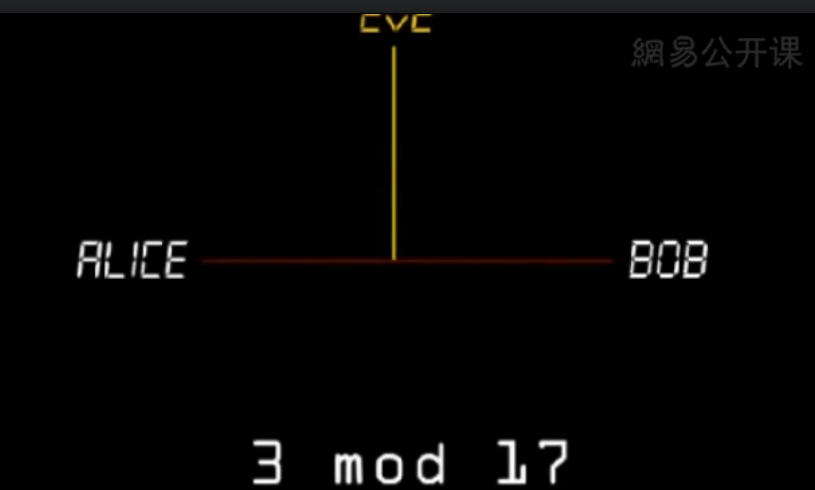$$3^{\color{red}{15}} \mathrm {mod} \ 17 \equiv {\color{blue} 6}$$

Bob选择一个私有数13，计算
$$3^{\color{red}{13}} \mathrm {mod} \ 17 \equiv {\color{blue} {12}}$$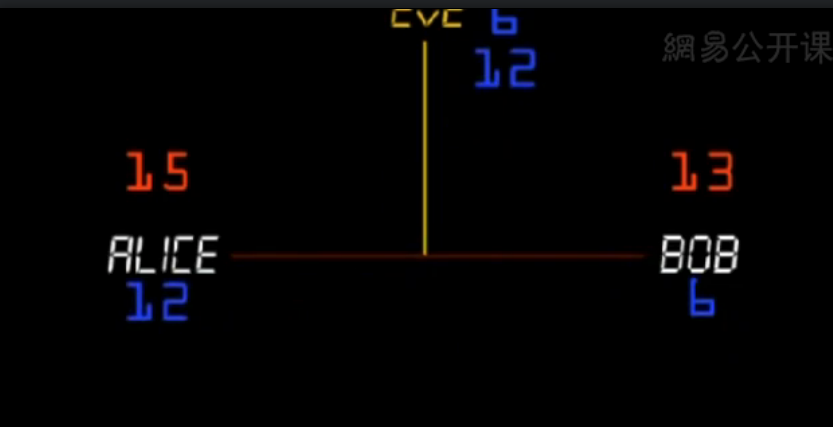Alice经过以下计算，得到共享密钥 (shared secret) ${\color{Apricot}{10} }$

$${\color{blue}{12} }^{\color{red}{15} } \mathrm {mod} \ 17 \equiv {\color{Apricot}{10} }$$

Bob也计算得到共享密钥，两者计算结果是相同的。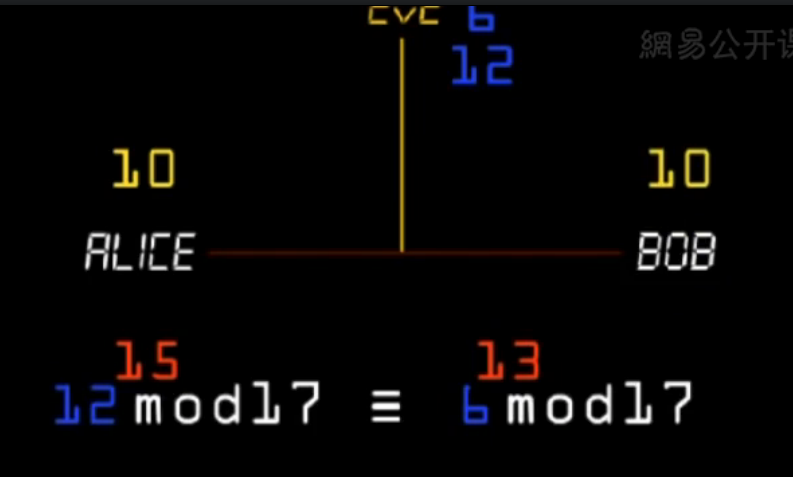$$3^{\color{red}{13 ^{15}} } \mathrm {mod} \ 17 \equiv 3^{\color{red}{15 ^{13}} } \mathrm {mod} \ 17$$

Eve将无法求出结果，没太看懂。

• 这两个私有数字，只要有一个就能解密吗？
• 17，3 是怎么达成一致的？传输过程被截获呢？是public的吗？

# RSA加密第一步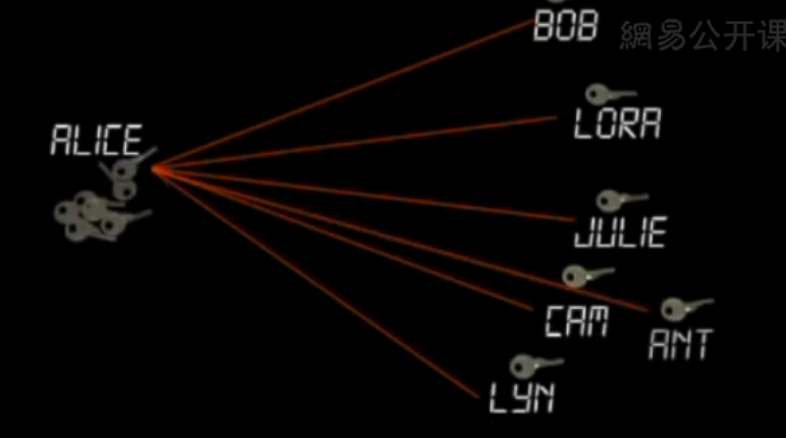1970年，英国数学家James Ellis试图实现公开密钥加密(non-secret encryption)。

Ellis并未找到相关的数学方法，但他提出了应该怎样做的思路。关键在于将密钥分为两个部分，一部分是加密密钥，一部分是解密密钥。解密密钥用于解密这一逆操作，而加密是通过加密密钥进行的。

# 扩展阅读

• 现代密码学 | 可汗学院
• http://www.ruanyifeng.com/blog/2013/06/rsa_algorithm_part_one.html